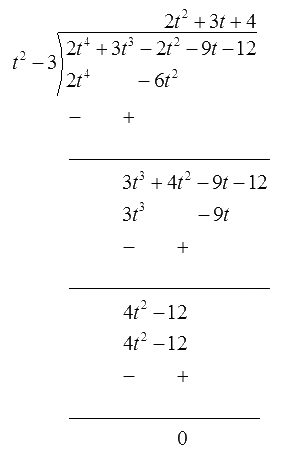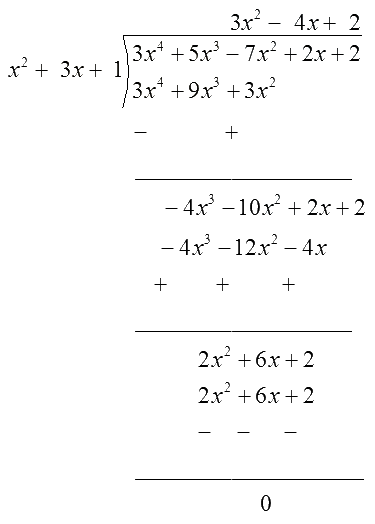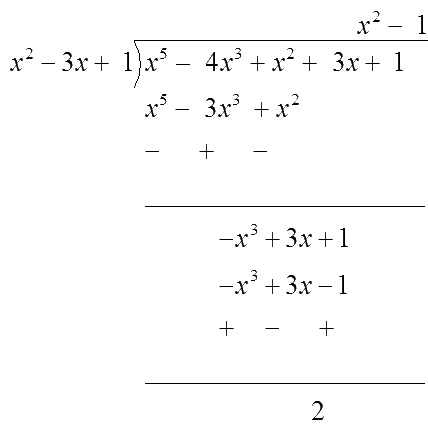# Ex.2.3 Q2 Polynomials Solution - NCERT Maths Class 10

Go back to  'Ex.2.3'

## Question

Check whether the first polynomial is a factor of the second polynomial by dividing the second polynomial by the first polynomial:

(i)

\begin{align} & {{t}^{2}}-3,\\ & 2{{t}^{4}}+3{{t}^{3}}-2{{t}^{2}}-9t-12\end{align}

(ii)

\begin{align}&{{x}^{2}}+3x+1, \\&3{{x}^{4}}+5{{x}^{3}}-7{{x}^{2}}+2x+2 \end{align}

(iii)

\begin{align}&{{x}^{3}}-3x+1,\\&{{x}^{5}}-4{{x}^{3}}+{{x}^{2}}+3x+1 \\ \end{align}

Video Solution
Polynomials
Ex 2.3 | Question 2

## Text Solution

What is unknown?

Whether the first polynomial is a factor of the second polynomial by dividing the second polynomial by the first polynomial.

Reasoning:

To solve this question, follow the same procedure given in question no $$1,$$ you have to observe only one thing - if the remainder is $$0$$ then the first polynomial is a factor of the second polynomial.

Steps:

(i)

\begin{align}\quad{{t}^{2}}-3,\;2{{t}^{4}}+3{{t}^{3}}-2{{t}^{2}}-9t-12\end{align}

\begin{align}{ {t^2} - 3 = {t^2} + 0.t - 3}\end{align}Since, the remainder is zero, $${t^2}-3$$ is a factor of $$2{t^4} + 3{t^3} - 2{t^2} - 9t - 12$$

(ii)

\begin{align}&{{x}^{2}}+3x+1, \\&3{{x}^{4}}+5{{x}^{3}}-7{{x}^{2}}+2x+2 \end{align}Quotient $$=3{{x}^{2}}-4x+2,$$   Remainder $$=0$$

Since, the remainder is zero, $${x^2} +3x +1$$  is a factor of $$3{x^4} + 5{x^3}-7{x^2} + 2x +2$$

(iii)

\begin{align}&{{x}^{3}}-3x+1,\\&{{x}^{5}}-4{{x}^{3}}+{{x}^{2}}+3x+1 \\ \end{align}Quotient $$={{x}^{2}}-1,$$   Remainder $$=2$$

Since, the remainder is not  zero, $${x^3}-3x + 1$$ is not a factor of $${x^5}-4{x^3} + {x^2} + 3x + 1$$

Learn from the best math teachers and top your exams

• Live one on one classroom and doubt clearing
• Practice worksheets in and after class for conceptual clarity
• Personalized curriculum to keep up with school